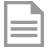# Avaliação de modelos volumétricos para plantio comercial de Calophyllum brasiliense Cambess na região sul do estado do Tocantins.pdf

## Rigorous cubing is the measurement of various diameters along the tree trunk to obtain the true volume of a single tree. To estimate a volume for a given planting, volumetric equations are used, which generate the volume with the least error and closest to the actual volume. Thus, rigorous cubing by the Smalian method was performed on 46 trees, determined by diameter class, with a minimum adopted diameter of 2 cm. Nine volumetric equations were tested, based on the following criteria: Adjusted coefficient of determination (R²aj), coefficient of variation in percentage (CV %), and graphical analysis of residues. With the data analysis, the best fit model was Schumacher-Hall, presenting the following results: adjusted R² = 0.979, CV% of 2.24, the graphical analysis of residual dispersion between the observed and estimated values showed that the function ln(v) = -15.0053 + 1.29298.ln(d) + 1.266519.ln(h) presented adequate adjustment. With the selected equation, the volume was estimated at 6.22 m³.ha-1 year, cons

Created by Marcos Giongo 2020-07-13T15:53:54.313-03:00
Modified by Marcos Giongo 2020-07-13T16:20:19.309-03:00
Versão atual: 1.0
Versões anteriores: